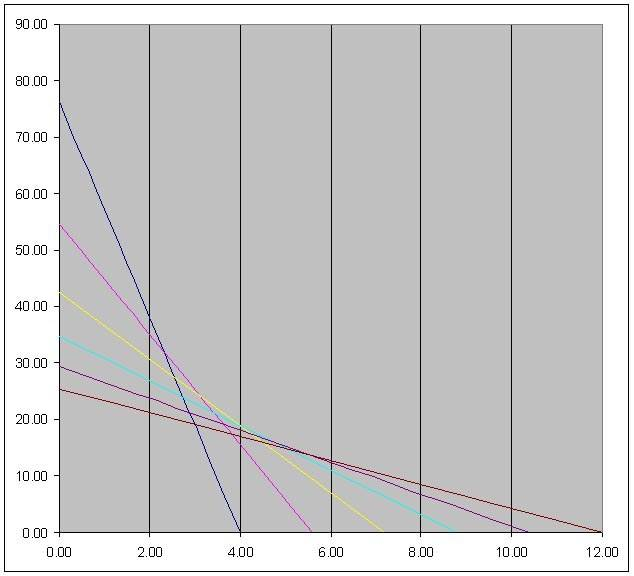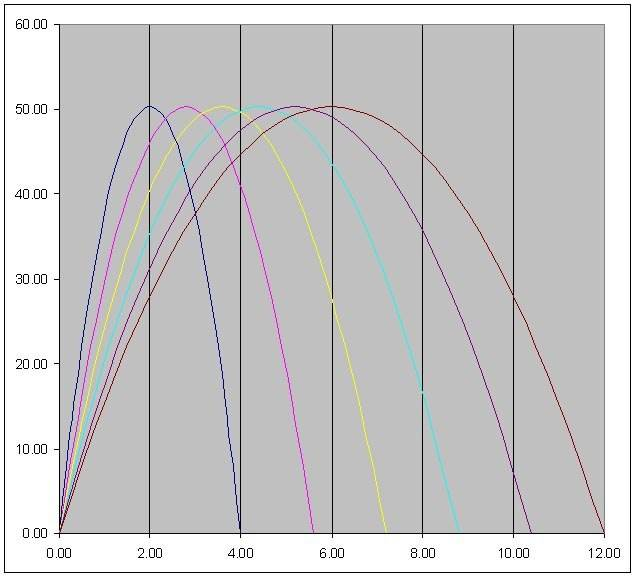# Calculating acceleration on different gears in vehicle

Nugatory
Mentor
But its not so simple. Take in mind i can change torque to anything i want using gears. I could apply a gearing that would make from 100 Nm of torque at engine a 50000 Nm of torque at wheels. That can't mean i could accelerate fast as hell, there is no free energy

You can get a very high acceleration that way, limited only because if you apply enough torque, something will break. However, a very high acceleration doesn't mean a very high final speed, not unless you can keep applying the torque for long enough to build up the speed.

Suppose you have an engine that delivers 100 Nm of torque at the crankshaft when operating at 6000 RPM at full throttle. You can gear it down to deliver 50000 Nm at the wheels, but then the wheels will only be turning at 12 RPM; for reasonable-sized wheels with a circumference of two meters that's about 1.4 km/hr. That's a bulldozer, something that can pull very hard but doesn't cover a lot of ground.

If it's light enough, its zero-to-1.4 acceleration will be pretty impressive. But if you want to use that impressive acceleration to win a drag race you'll need to either find a way of getting the motor to deliver the same torque at much higher rev rates (there's a reason why Formula 1 engines do more than 15000 RPM, and aircraft turbines spin even faster) or get the motor to deliver more torque at 6000 RPM so that you can get the same impressive acceleration with less aggressive gearing. Either way, you're asking the motor to generate more power (the product of torque and revs per unit time).

Nugatory
Mentor
But what is "distance covered by the force",
and what is difference when force of 100N apply on 70kg milf over distance of one metre in one second than when force of 100N apply on 70kg milf over distance of two metres in one second ?

You're trying to specify the distance, the time, the force, and the mass all at once, and you can't do that because they're connected by Newton's laws. For example, the force and the mass determine the acceleration and the acceleration and the initial speed determine the time it takes to cover one meter; so once you've chosen a mass and a force, you're stuck with a particular time.

If you don't mind, I'm going to do this with a mass of 50 kg instead of 70, because I'm doing the arithmetic in my head.

Say the 50 kg mass starts at rest, and we apply 100N to it for a distance of one meter. The total work done is 100 Joules; this comes from ##W=Fd##. All this work ends up as kinetic energy of the mass and I can use the kinetic energy formula ##E=\frac{mv^2}{2} \Rightarrow v=\sqrt{2E/m}## to calculate that the mass is moving at a speed of ##2 m/sec## after it's covered the first meter. Its acceleration (from ##F=ma##) is ##2 m/sec^2## so I can calculate (from ##s=\frac{at^2}{2}##) that it took one second to cover that one meter distance. The power output was 100 Joules over a period of one second, which is 100 Watts.

There are two important points here:
1) That 100 watts is an average over the entire second. At the start of the second when the speed was zero, the instaneous power delivered was zero, and by the end of the second it was 200 watts. So if my motor wasn't capable of delivering 200 watts, it wouldn't have been able to keep applying the full 100N of force for the entire second.
2) if I'm going to continue applying 100N to the mass for another second, thereby maintaining the same ##2 m/sec^2## acceleration so that it is moving at ##4 m/sec##, I'll need an even more powerful motor. Don't take my word for it, calculate it... The work done in the second meter is the same (##W=Fd##) but the time it takes the mass to cover that meter is less because it's moving faster.

rcgldr
Homework Helper
I made up some gear ratios for the motor shown in the first post to create a pair of graphs. The first graph shows driven wheel torque (or force if radius is 1 meter) versus speed for each gear, and the second graph shows driven wheel power versus speed for each gear. The ideal shift points occur where the lines cross (the lines cross at the same speeds in both graphs).Last edited: